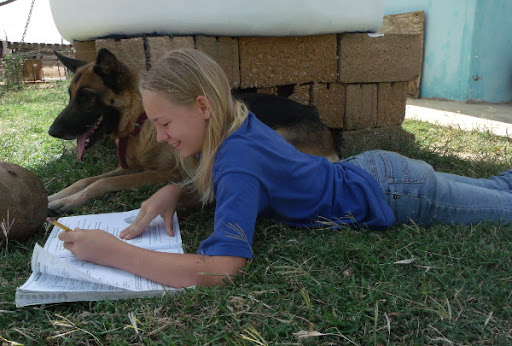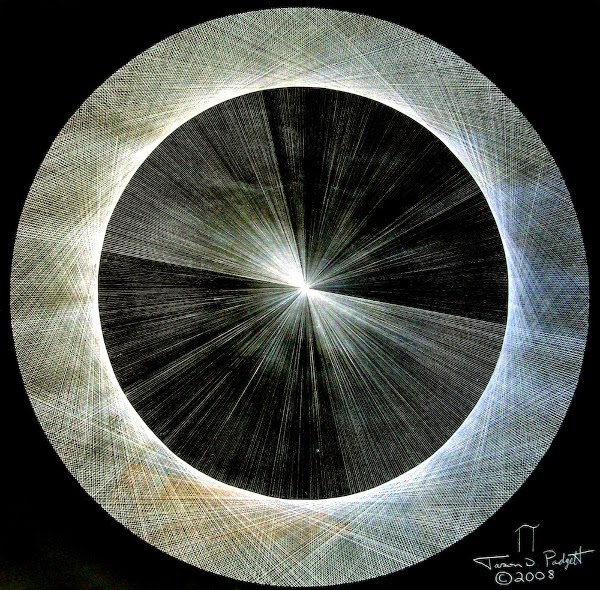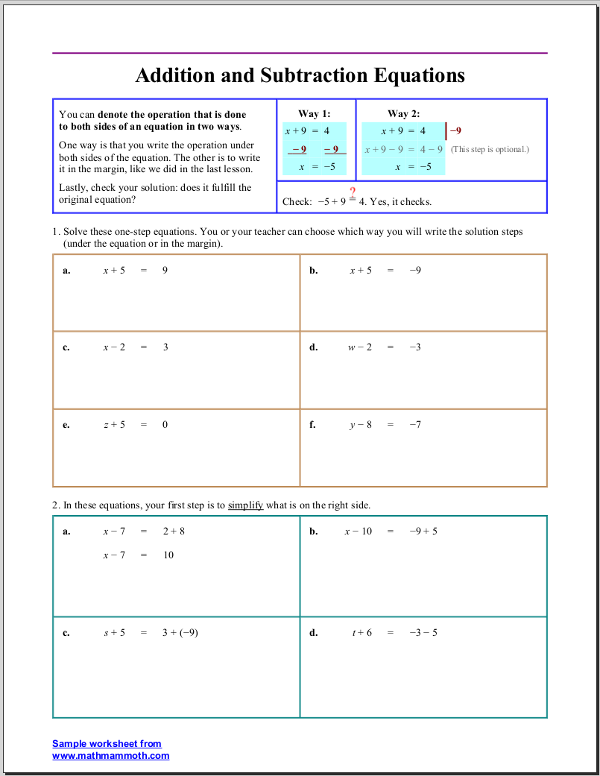## Posts

Showing posts from June, 2014

### Worksheets for simplifying expressionsI've made a worksheet generator for simplifying expressions , meant for pre-algebra and algebra 1. The expressions include ones where you need to combine like terms (such as 2t − 9 − 6t + 2), use the distributive property (such as 9 − 2(x + 7)), and to multiply and divide monomials, such as 2x 2 · (−5x 3 ) and −4x 2 · y 2 / 3x 5 . To customize the worksheets, you can control the number of problems, difficulty level, range of numbers used as coefficients and constants, the usage of decimals, the amount of workspace, a border around the problems, and additional instructions.

### Math Mammoth Photo Contest### Brain Injury Turns Man Into Math GeniusI found this very interesting... a brain injury 'unlocked' a part of Jason Padgett's brain, and now the man sees geometric shapes and angles everywhere! http://www.livescience.com/45349-brain-injury-turns-man-into-math-genius.html See also a gallery of his drawings . The one below is a circle (or two) created by drawing many triangles. He says, "I drew circles out of 180 triangles, then 360, then 720." With a finer pencil, he could draw even more. "Through this process, I came to understand how pi is calculated by measuring the area of a circle."